Next: Artificial Galaxy: Full Matrix Up: Restrictions on the Scenario Previous: Binary Pulsar Diversity and

## X-ray Galactic Sources and Binary Radiopulsars as Probes of the Initial Mass Ratio f(q) and Kick Velocity w

Now we present the topographical map for the COOC criterion calculated for both galactic X-ray sources taken from Table 4 and binary pulsar species from Table 6 in coordinates the initial mass ratio exponent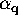- mean kick velocity(Figure 26). The calculations were performed for both the maxwellian (equation 7.4.1) - (left-hand panel) and Lyne and Lorimer's (equation 7.4.2)  - (right-hand panel) distributions for the kick velocity w. The innermost curve corresponds to a half-an-order uncertainty between observations and calculations (COOC = 3.3).

As we have shown just above, the binary pulsars' statistics favor lower kick velocities, whereas Galactic X-ray luminosity acts in the opposite direction. This largely defines the shape of the curves in Figure 26. A wide kick velocity distribution (like Lyne and Lorimer's) allows a wider region of parametersand w for the same comparison level between observations and calculations. If one takes Lyne and Lorimer's kick withkm s, the feasible initial mass ratio exponent can range from0 to 1.5 within a seven times uncertainty between the observations and calculations.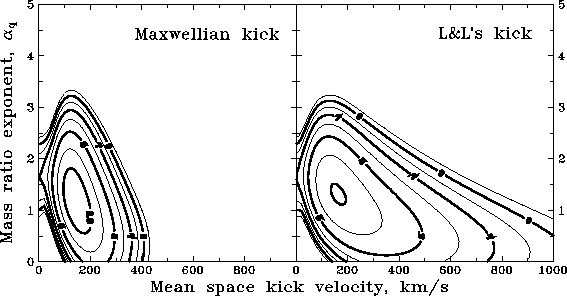Figure 26: The COOC criterion for X-ray galactic sources and binary radiopulsars versus the initial mass ratio exponentand mean space kick velocity for different kick velocity distributions.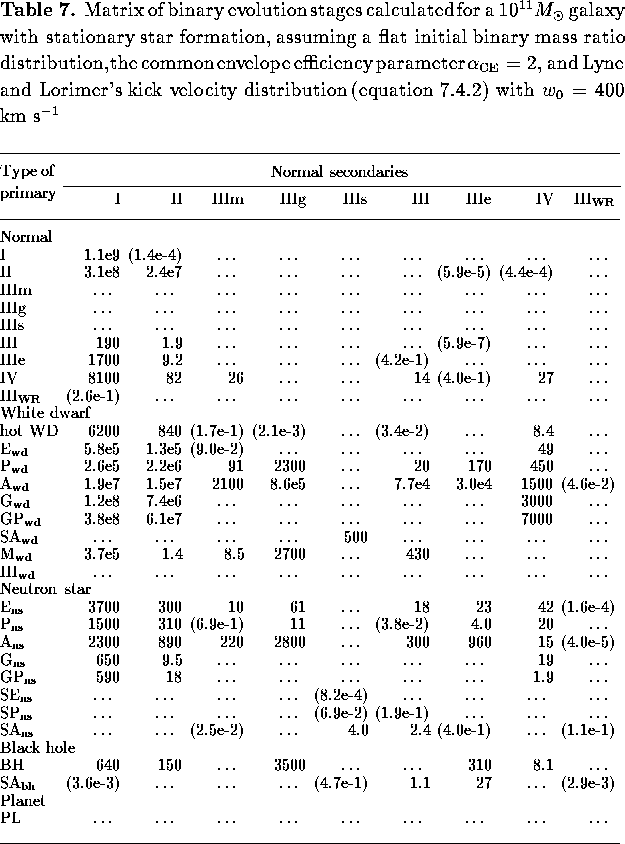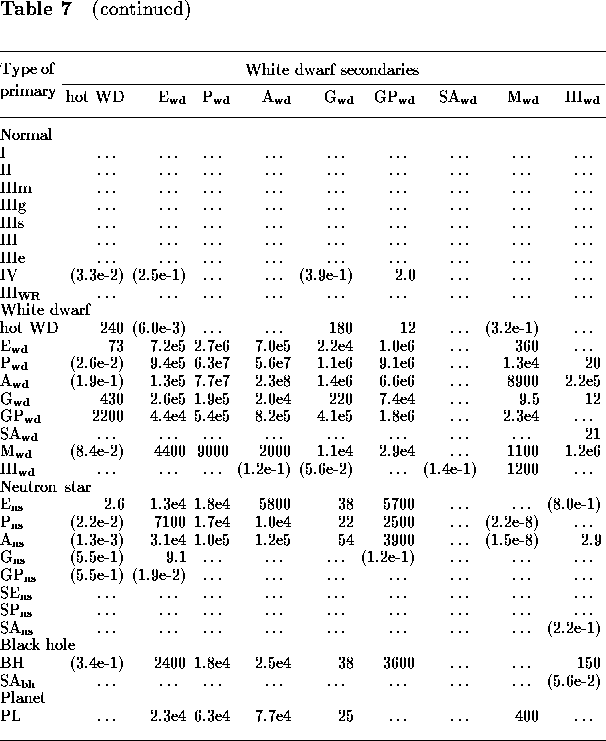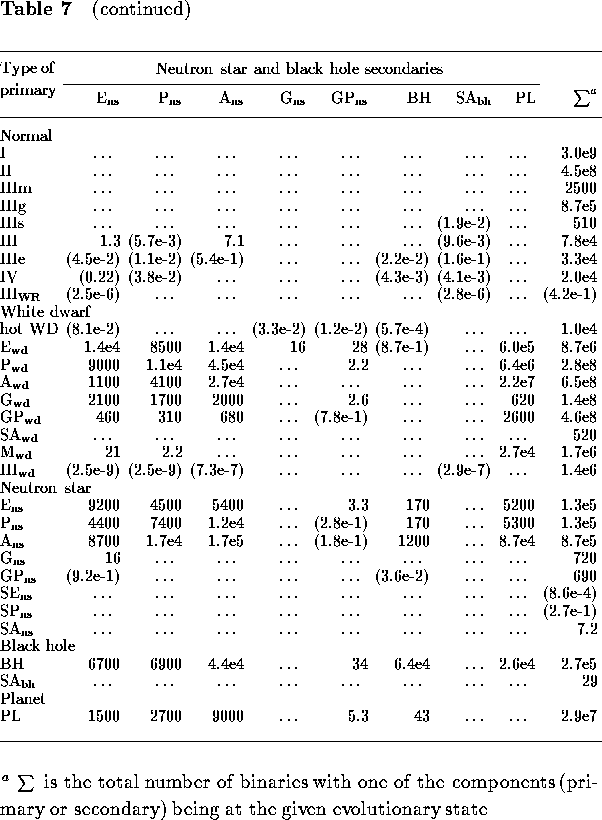Mike E. Prokhorov
Sat Feb 22 18:38:13 MSK 1997